University Physics Volume 2

72.

A vector field $E→E→$ (not necessarily an electric field; note units) is given by $E→=3x2k^.E→=3x2k^.$ Calculate $∫SE→·n^da,∫SE→·n^da,$ where S is the area shown below. Assume that $n^=k^.n^=k^.$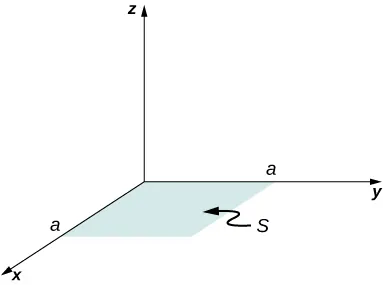73.

Repeat the preceding problem, with $E→=2xi^+3x2k^.E→=2xi^+3x2k^.$

74.

A circular area S is concentric with the origin, has radius a, and lies in the yz-plane. Calculate $∫SE→·n^dA∫SE→·n^dA$ for $E→=3z2i^.E→=3z2i^.$

75.

(a) Calculate the electric flux through the open hemispherical surface due to the electric field $E→=E0k^E→=E0k^$ (see below). (b) If the hemisphere is rotated by $90°90°$ around the x-axis, what is the flux through it?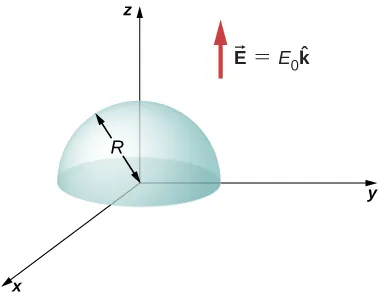76.

Suppose that the electric field of an isolated point charge were proportional to $1/r2+σ1/r2+σ$ rather than $1/r2.1/r2.$ Determine the flux that passes through the surface of a sphere of radius R centered at the charge. Would Gauss’s law remain valid?

77.

The electric field in a region is given by $E→=a/(b+cx)i^,E→=a/(b+cx)i^,$ where $a=200N·m/C,a=200N·m/C,$$b=2.0m,b=2.0m,$ and $c=2.0.c=2.0.$ What is the net charge enclosed by the shaded volume shown below?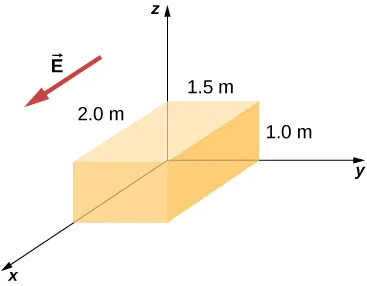78.

Two equal and opposite charges of magnitude Q are located on the x-axis at the points +a and –a, as shown below. What is the net flux due to these charges through a square surface of side 2a that lies in the yz-plane and is centered at the origin? (Hint: Determine the flux due to each charge separately, then use the principle of superposition. You may be able to make a symmetry argument.)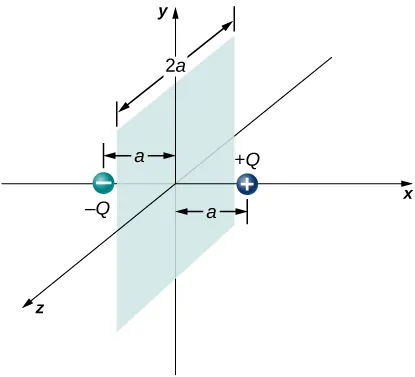79.

A fellow student calculated the flux through the square for the system in the preceding problem and got 0. What went wrong?

80.

A $10cm×10cm10cm×10cm$ piece of aluminum foil of 0.1 mm thickness has a charge of $20μC20μC$ that spreads on both wide side surfaces evenly. You may ignore the charges on the thin sides of the edges. (a) Find the charge density. (b) Find the electric field 1 cm from the center, assuming approximate planar symmetry.

81.

Two $10cm×10cm10cm×10cm$ pieces of aluminum foil of thickness 0.1 mm face each other with a separation of 5 mm. One of the foils has a charge of $+30μC+30μC$ and the other has $−30μC−30μC$. (a) Find the charge density at all surfaces, i.e., on those facing each other and those facing away. (b) Find the electric field between the plates near the center assuming planar symmetry.

82.

Two large copper plates facing each other have charge densities $±4.0C/m2±4.0C/m2$ on the surface facing the other plate, and zero in between the plates. Find the electric flux through a $3cm×4cm3cm×4cm$ rectangular area between the plates, as shown below, for the following orientations of the area. (a) If the area is parallel to the plates, and (b) if the area is tilted $θ=30°θ=30°$ from the parallel direction. Note, this angle can also be $θ=180°+30°.θ=180°+30°.$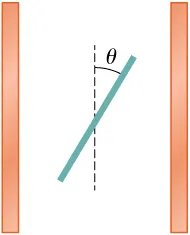83.

The infinite slab between the planes defined by $z=−a/2z=−a/2$ and $z=a/2z=a/2$ contains a uniform volume charge density $ρρ$ (see below). What is the electric field produced by this charge distribution, both inside and outside the distribution?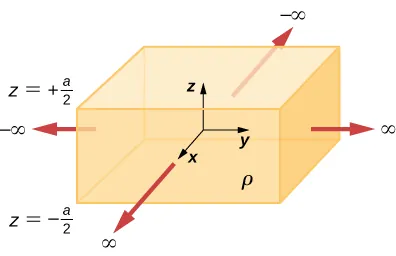84.

A total charge Q is distributed uniformly throughout a spherical volume that is centered at $O1O1$ and has a radius R. Without disturbing the charge remaining, charge is removed from the spherical volume that is centered at $O2O2$ (see below). Show that the electric field everywhere in the empty region is given by

$E → = Q r → 4 π ε 0 R 3 , E → = Q r → 4 π ε 0 R 3 ,$

where $r→r→$ is the displacement vector directed from $O1toO2.O1toO2.$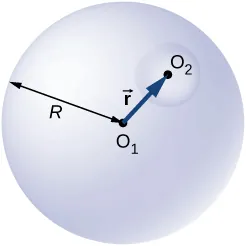85.

A non-conducting spherical shell of inner radius $a1a1$ and outer radius $b1b1$ is uniformly charged with charged density $ρ1ρ1$ inside another non-conducting spherical shell of inner radius $a2a2$ and outer radius $b2b2$ that is also uniformly charged with charge density $ρ2ρ2$. See below. Find the electric field at space point P at a distance r from the common center such that (a) $r>b2,r>b2,$ (b) $a2 (c) $b1 (d) $a1 and (e) $r.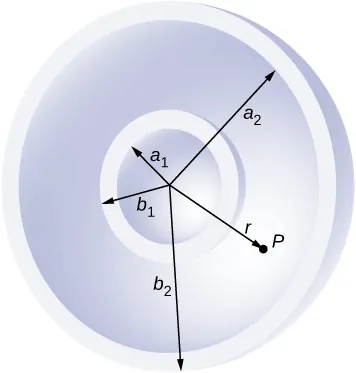86.

Two non-conducting spheres of radii $R1R1$ and $R2R2$ are uniformly charged with charge densities $ρ1ρ1$ and $ρ2,ρ2,$ respectively. They are separated at center-to-center distance a (see below). Find the electric field at point P located at a distance r from the center of sphere 1 and is in the direction $θθ$ from the line joining the two spheres assuming their charge densities are not affected by the presence of the other sphere. (Hint: Work one sphere at a time and use the superposition principle.)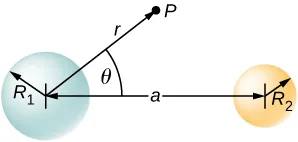87.

A disk of radius R is cut in a non-conducting large plate that is uniformly charged with charge density $σσ$ (coulomb per square meter). See below. Find the electric field at a height h above the center of the disk. $(h>>R,h<>R,h< (Hint: Fill the hole with $±σ.)±σ.)$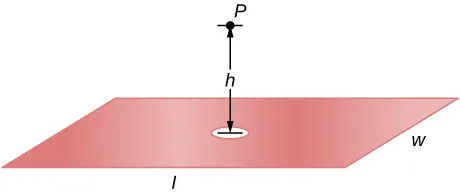88.

Concentric conducting spherical shells carry charges Q and –Q, respectively (see below). The inner shell has negligible thickness. Determine the electric field for (a) $r (b) $a (c) $b and (d) $r>c.r>c.$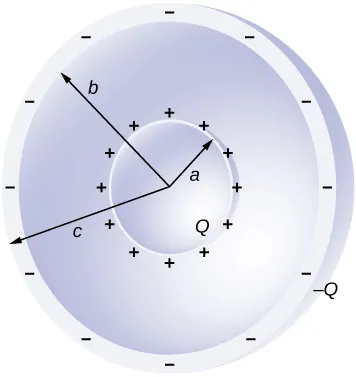89.

Shown below are two concentric conducting spherical shells of radii $R1R1$ and $R2R2$, each of finite thickness much less than either radius. The inner and outer shell carry net charges $q1q1$ and $q2,q2,$ respectively, where both $q1q1$ and $q2q2$ are positive. What is the electric field for (a) $r (b) $R1 and (c) $r>R2?r>R2?$ (d) What is the net charge on the inner surface of the inner shell, the outer surface of the inner shell, the inner surface of the outer shell, and the outer surface of the outer shell?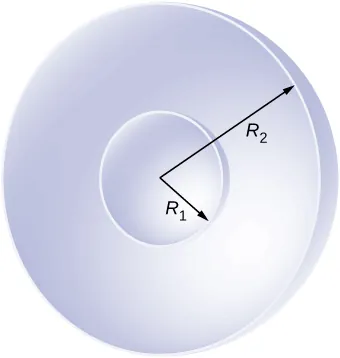90.

A point charge of $q=5.0×10−8Cq=5.0×10−8C$ is placed at the center of an uncharged spherical conducting shell of inner radius 6.0 cm and outer radius 9.0 cm. Find the electric field at (a) $r=4.0cmr=4.0cm$, (b) $r=8.0cmr=8.0cm$, and (c) $r=12.0cmr=12.0cm$. (d) What are the charges induced on the inner and outer surfaces of the shell?

Order a print copy

As an Amazon Associate we earn from qualifying purchases.